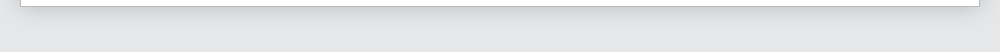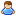Login Products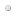SalesSupportDownloadsAbout
 Home » Technical Support » Elevate Web Builder Technical Support » Support Forums » Elevate Web Builder General » View Thread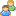View Thread

The following is the text of the current message along with any replies.
 Messages 1 to 5 of 5 total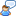Double value display format
 Wed, Apr 5 2017 3:04 AM Permanent Link kentersoft Hi,  In TEdit and TGrid Control, how to set a Money value's display format like 12,345.67  Is there have some function like Delphi Format function. Thanks. Wed, Apr 5 2017 12:18 PM Permanent Link Howard Chandler Fiscalsoft Corporation On 04/05/2017 3:04 AM, kentersoft wrote: > Hi, >   In TEdit and TGrid Control, how to set a Money value's display format like 12,345.67 >   Is there have some function like Delphi Format function. > > Thanks. > Hi, To my knowledge, there isn't such a function. It would be very nice to have one eventually. A TMaskEdit would also be helpful with formatting data. I haven't used a TGrid(yet); only TEdits so far. What I've done is to use the TEdit control's OnKeyPress and OnExit events. The KeyPress event controls the allowable characters in the edit box (EditGross): function TFrmMainForm.EditGrossKeyPress(Sender: TObject; Key: Char; ShiftKey, CtrlKey, AltKey: Boolean): Boolean; begin  TEdit(Sender).Error := False;  if (Key >= '0' and Key <= '9') or (Key = '.') then   result := True  else  begin   TEdit(Sender).Error := True;   result := False;  end; end; If you are allowing negative numbers, then also add: or (Key = '-') to the if statement above. In the OnExit event, I format the Text: procedure TFrmMainForm.EditGrossExit(Sender: TObject); var  ProperValue: Double; begin  // Ensure 2 decimal places with FloatToStr  TEdit(Sender).Error := False;  try   ProperValue := StrToFloat(TEdit(Sender).Text);   TEdit(Sender).Text := FloatToStr(ProperValue,2);  except   TEdit(Sender).Error := True;   TEdit(Sender).Setfocus;  end; end; This seems to work well for me. I'm just now getting into EWB, so there are probably better ways to do what I'm doing. Maybe one of the gurus will tell us both a better way. HTH, Howard Wed, Apr 5 2017 2:10 PM Permanent Link Walter Matte Tactical Business Corporation Add the Comma  ... function TForm1.AddThousandSeparator(s : string): string; var    LS, L2, I, N, D: Integer;    Temp : string;    sDec : string; begin    d := pos('.',s);  // Check for Decumal    if d > 0 then    begin      sDec :=  copy(s,d,length(s) - (d-1));      s := copy(s,1,d-1);    end    else      sDec := '';    result := S ;    LS := Length (S);    N := 1 ;    if LS > 1 then    begin        if S  = '-' then  // check for negative value        begin            N := 2 ;            LS := LS - 1 ;        end ;    end ;    if LS <= 3 then exit ;    L2 := (LS - 1) div 3;    Temp := '';    for I := 1 to L2 do         Temp := ',' + Copy (S, LS - 3 * I + 1, 3) + Temp;    Result := Copy (S, N, (LS - 1) mod 3 + 1) + Temp;    if N > 1 then Result := '-' + Result ;    Result := Result + sDec; end; // Usage  edit1.Text := AddThousandSeparator(FloatToStr(2134.3,2)); Walter Wed, Apr 5 2017 3:47 PM Permanent Link Trinione This is what I use. ---------------------------------------------- function AddThousandSeparator(s: string): string; var  i, j, startInsPos: Integer;  ins: integer;   // number of commas required  sCents, sDollars: string;  sl: array of string;   begin          try    s := ReverseString(s);    startInsPos := 3;      sCents := Copy(s, 1, 3);    sDollars := Copy(s, 4);    sl := Split(sDollars, '');      ins := 0;    if Length(sl) > 3 then    begin      // Only concerned with characters over character position 3 as that is when commas are needed      ins := (Length(sl)-3) div 3;        // If there isn't a modular remainder, add 1 to number of comma insertions required      // Eg. If sl is '4321' then comma insertion value = 0.      // Length(sl)-3 mod 3, equals 1, so it now has a value to use in the for loop,      // if no modular remainder it just keeps the insertion value as is.if ((Length(sl)-3) mod 3 <> 0) then        Inc(ins);        j := 0;      for i := 1 to ins do      begin        Insert(',', sl, i*3+j);        Inc(j);      end;    end;  {    if (Length(sl) >= 4) And (Length(sl) <= 7) then      Insert(',', sl, 3);      if (Length(sl) >= 8) And (Length(sl) <= 11) then      Insert(',', sl, 7);      if (Length(sl) >= 12) then      Insert(',', sl, 11);  }    result := ReverseString(sCents + Join(sl));  except    result := '';  end; end; //function ShowAmountCurrency(amt: double): string; function ShowAmountCurrency(amt: string): string; begin  try    amt := FloatToStr(StrToFloat(amt), 2);      result := AddThousandSeparator(amt);  except    result := '';  end; end; kentersoft wrote: Hi,  In TEdit and TGrid Control, how to set a Money value's display format like 12,345.67  Is there have some function like Delphi Format function. Thanks. Thu, Apr 6 2017 3:17 AM Permanent Link kentersoft thank you all very much, since i have many TEdit and TGrid to set, I will let it as just now, if have a offical Solution will be great.